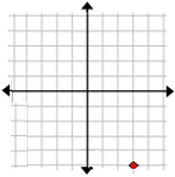# Naming the quadrant or axis of a point given its graph Online Quiz

Following quiz provides Multiple Choice Questions (MCQs) related to Naming the quadrant or axis of a point given its graph. You will have to read all the given answers and click over the correct answer. If you are not sure about the answer then you can check the answer using Show Answer button. You can use Next Quiz button to check new set of questions in the quiz.Q 1 - Name the quadrant or axis of the point in the following graph.### Explanation

Step 1 − The point in the graph has coordinates (2, −2).

Step 2 − It lies in the quadrant 4.

Q 2 - Name the quadrant or axis of the point in the following graph.### Explanation

Step 1 − The point in the graph has coordinates (−2, 2).

Step 2 − It lies in the quadrant 2.

Q 3 - Name the quadrant or axis of the point in the following graph.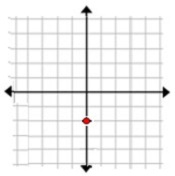### Explanation

Step 1 − The point in the graph has coordinates (0, −2).

Step 2 − It lies on the negative y axis.

Q 4 - Name the quadrant or axis of the point in the following graph.### Explanation

Step 1 − The point in the graph has coordinates (0, 3).

Step 2 − It lies on the positive y axis.

Q 5 - Name the quadrant o r axis of the point in the following graph.### Explanation

Step 1 − The point in the graph has coordinates (−3, 0).

Step 2 − It lies on the negative x axis.

Q 6 - Name the quadrant or axis of the point in the following graph.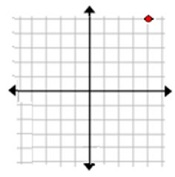### Explanation

Step 1 − The point in the graph has coordinates (4, 5).

Step 2 − It lies in the quadrant 1.

Q 7 - Name the quadrant or axis of the point in the following graph.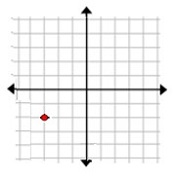### Explanation

Step 1 − The point in the graph has coordinates (−3, −2).

Step 2 − It lies in the quadrant 3.

Q 8 - Name the quadrant or axis of the point in the following graph.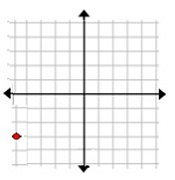### Explanation

Step 1 − The point in the graph has coordinates (−5, −3)

Step 2 − It lies in the quadrant 3.

Q 9 - Name the quadrant or axis of the point in the following graph.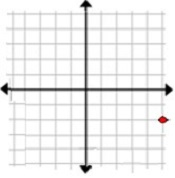### Explanation

Step 1 − The point in the graph has coordinates (5, −2).

Step 2 − It lies in the quadrant 4.

Q 10 - Name the quadrant or axis of the point in the following graph.